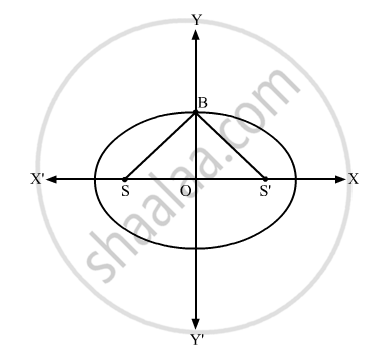Advertisement Remove all ads

# If S and S' Are Two Foci of the Ellipse X 2 a 2 + Y 2 B 2 = 1 and B is an End of the Minor Axis Such that ∆Bss' is Equilateral, Then Write the Eccentricity of the Ellipse. - Mathematics

If S and S' are two foci of the ellipse $\frac{x^2}{a^2} + \frac{y^2}{b^2} = 1$ and B is an end of the minor axis such that ∆BSS' is equilateral, then write the eccentricity of the ellipse.

Advertisement Remove all ads

#### Solution$\text{ We know that the focal distance of a point B }(0, b) \text{ is } a \pm e . 0 = a$
$\text{ i . e } . SB = SB' = a$
therefore SB + S B^' = 2a
"Since" ∆ {BSS}^' \"is equilateral, we have":
SB = S S^' = S^' B = 2ae
$\Rightarrow 2ae + 2ae = 2a$
$\Rightarrow 4ae = 2a$
$\Rightarrow e = \frac{2}{4}$
$\Rightarrow e = \frac{1}{2}$

Is there an error in this question or solution?
Advertisement Remove all ads

#### APPEARS IN

RD Sharma Class 11 Mathematics Textbook
Chapter 26 Ellipse
Q 7 | Page 27
Advertisement Remove all ads
Advertisement Remove all ads
Share
Notifications

View all notifications

Forgot password?